# Encryption Session 9 INST 346 Simple encryption scheme

• Slides: 20
Download presentationEncryption Session 9 INST 346Simple encryption scheme substitution cipher: substituting one thing for another § monoalphabetic cipher: substitute one letter for another plaintext: abcdefghijklmnopqrstuvwxyz ciphertext: e. g. : mnbvcxzasdfghjklpoiuytrewq Plaintext: bob. i love you. alice ciphertext: nkn. s gktc wky. mgsbc Encryption key: mapping from set of 26 letters to set of 26 lettersStream and Block Ciphers § n substitution ciphers, M 1, M 2, …, Mn § cycling pattern: • e. g. , n=4: M 1, M 3, M 4, M 3, M 2; . . • random initialization § for each new plaintext symbol, use subsequent substitution pattern in cyclic pattern • dog: d from M 1, o from M 3, g from M 4 Encryption key: n substitution ciphers, and cyclic patternAES: Advanced Encryption Standard § symmetric-key NIST standard, replaced DES (Nov 2001) § processes data in 128 bit blocks § 128, 192, or 256 bit keys § brute force decryption (try each key) taking 1 sec on DES, takes 149 trillion years for AES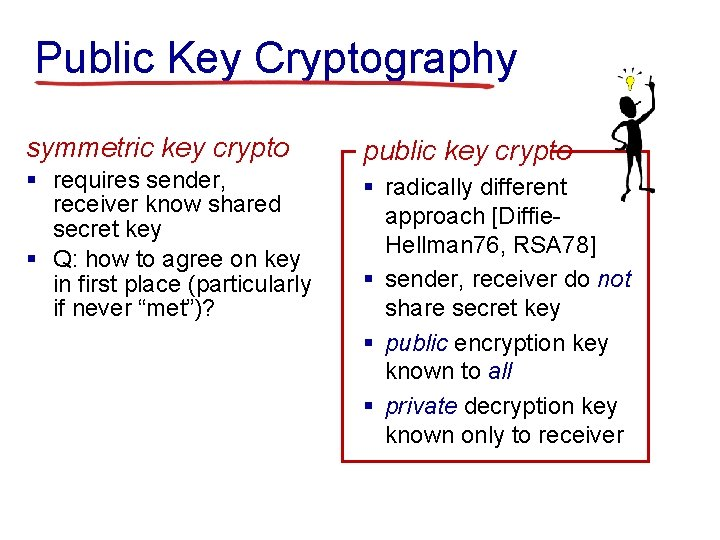Public Key Cryptography symmetric key crypto public key crypto § requires sender, receiver know shared secret key § Q: how to agree on key in first place (particularly if never “met”)? § radically different approach [Diffie. Hellman 76, RSA 78] § sender, receiver do not share secret key § public encryption key known to all § private decryption key known only to receiverPublic key cryptography + Bob’s public B key K K plaintext message, m encryption algorithm ciphertext + B K (m) - Bob’s private B key decryption algorithm plaintext message + m = KB (K (m)) B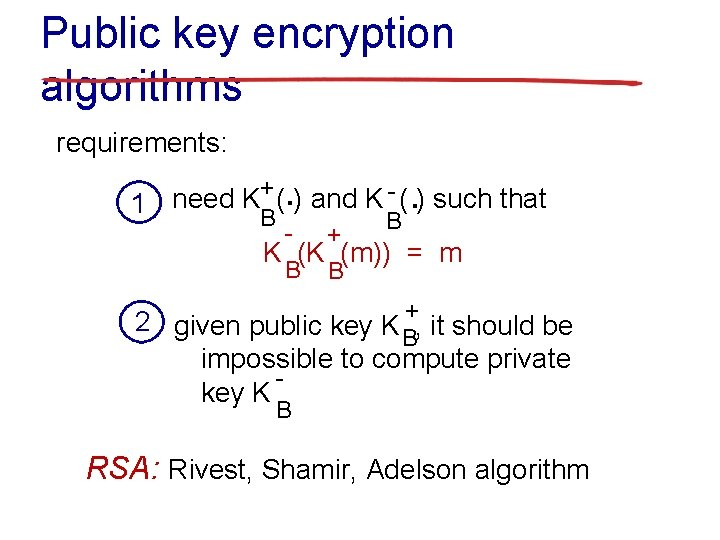Public key encryption algorithms requirements: . . B + ( ) and K - ( ) such that need K 1 B - + B B K (K (m)) = m 2 given public key K +, it should be B impossible to compute private key K B RSA: Rivest, Shamir, Adelson algorithm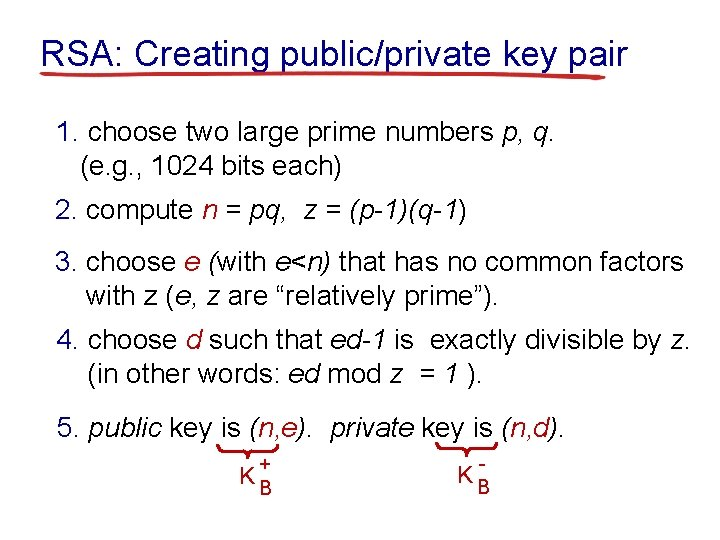RSA: Creating public/private key pair 1. choose two large prime numbers p, q. (e. g. , 1024 bits each) 2. compute n = pq, z = (p-1)(q-1) 3. choose e (with e<n) that has no common factors with z (e, z are “relatively prime”). 4. choose d such that ed-1 is exactly divisible by z. (in other words: ed mod z = 1 ). 5. public key is (n, e). private key is (n, d). + KB - KBRSA: encryption, decryption 0. given (n, e) and (n, d) as computed above 1. to encrypt message m (<n), compute c = me mod n 2. to decrypt received bit pattern, c, compute m = cd mod n d m = (me mod n) mod n cRSA example: Bob chooses p=5, q=7. Then n=35, z=24. e=5 (so e, z relatively prime). d=29 (so ed-1 exactly divisible by z). encrypting 8 -bit messages. encrypt: decrypt: bit pattern m me 0000 l 000 12 24832 c 17 c d 48196857210675091411825223071697 c = me mod n 17 m = cd mod n 12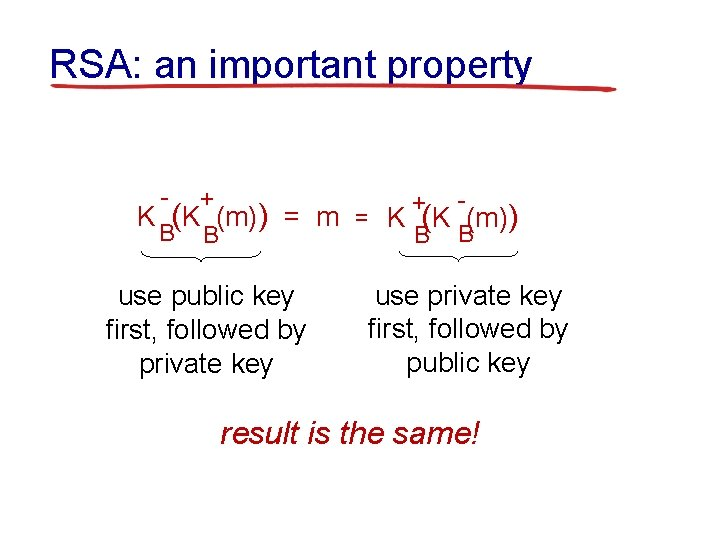RSA: an important property - + + K (K (m)) = m = K (K (m)) B B use public key first, followed by private key use private key first, followed by public key result is the same!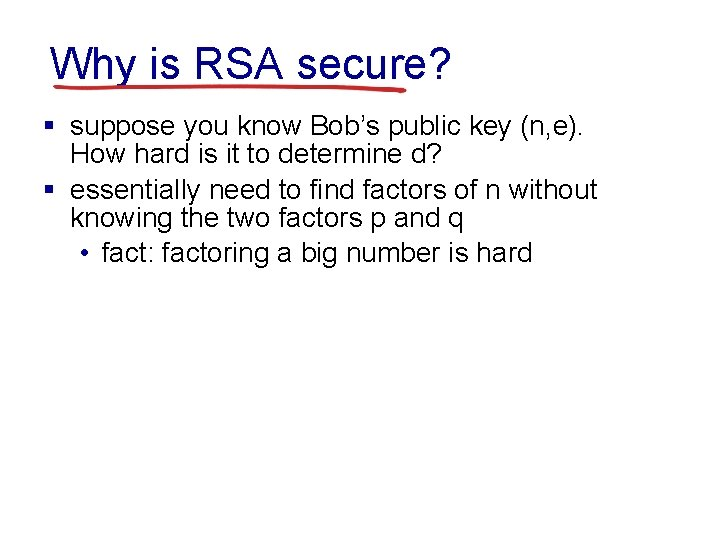Why is RSA secure? § suppose you know Bob’s public key (n, e). How hard is it to determine d? § essentially need to find factors of n without knowing the two factors p and q • fact: factoring a big number is hardRSA in practice: session keys § exponentiation in RSA is computationally intensive § DES is at least 100 times faster than RSA § use public key crypto to establish secure connection, then establish second key – symmetric session key – for encrypting data session key, KS § Bob and Alice use RSA to exchange a symmetric key KS § once both have KS, they use symmetric key cryptographyDigital signatures cryptographic technique analogous to handwritten signatures: § sender (Bob) digitally signs document, establishing he is document owner/creator. § verifiable, nonforgeable: recipient (Alice) can prove to someone that Bob, and no one else (including Alice), must have signed document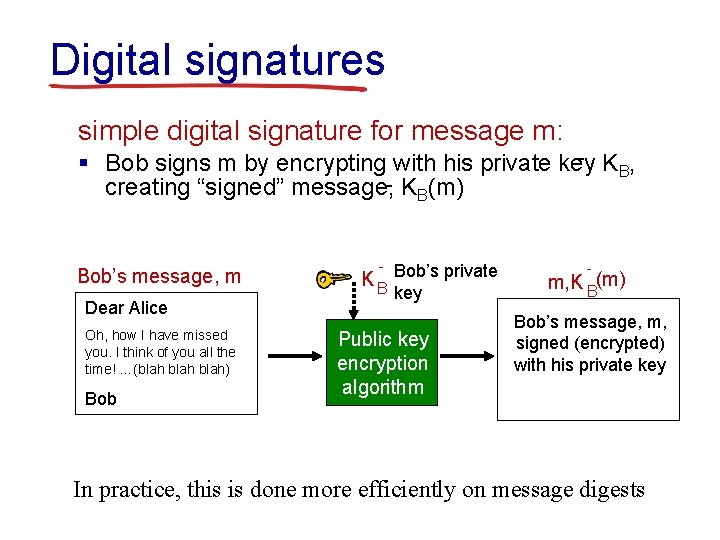Digital signatures simple digital signature for message m: - K , § Bob signs m by encrypting with his private key B creating “signed” message, - KB(m) Bob’s message, m Dear Alice Oh, how I have missed you. I think of you all the time! …(blah) Bob - Bob’s private KB key Public key encryption algorithm m, K B(m) Bob’s message, m, signed (encrypted) with his private key In practice, this is done more efficiently on message digests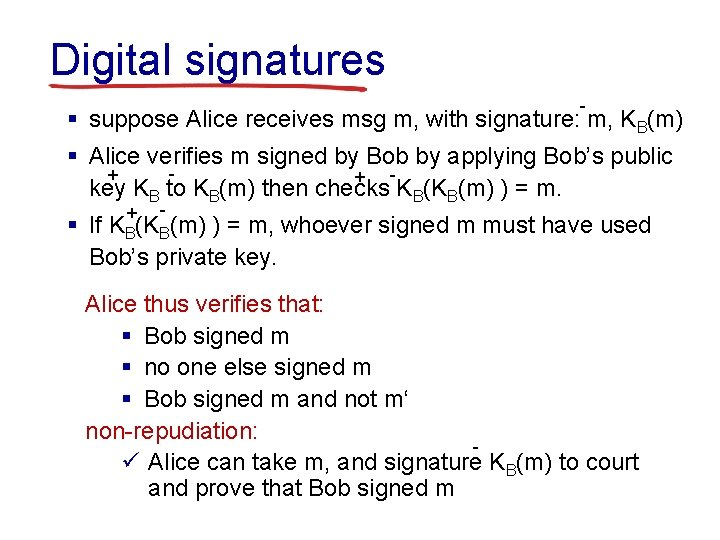Digital signatures - § suppose Alice receives msg m, with signature: m, KB(m) § Alice verifies m signed by Bob by applying Bob’s public + + key KB to KB(m) then checks KB(KB(m) ) = m. + - § If KB(KB(m) ) = m, whoever signed m must have used Bob’s private key. Alice thus verifies that: § Bob signed m § no one else signed m § Bob signed m and not m‘ non-repudiation: ü Alice can take m, and signature KB(m) to court and prove that Bob signed mMessage digests goal: fixed-length, easy- to-compute digital “fingerprint” § apply hash function H to m, get fixed size message digest, H(m). large message m H: Hash Function H(m) Hash function properties: § many-to-1 § produces fixed-size msg digest (fingerprint) § given message digest x, computationally infeasible to find m such that x = H(m)TCP checksum: poor crypto hash function Internet checksum has some properties of hash function: § produces fixed length digest (16 -bit sum) of message § is many-to-one But given message with given hash value, it is easy to find another message with same hash value: message IOU 1 00. 9 9 BOB ASCII format 49 4 F 55 31 30 30 2 E 39 39 42 D 2 42 B 2 C 1 D 2 AC message IOU 9 00. 1 9 BOB different messages but identical checksums! ASCII format 49 4 F 55 39 30 30 2 E 31 39 42 D 2 42 B 2 C 1 D 2 AC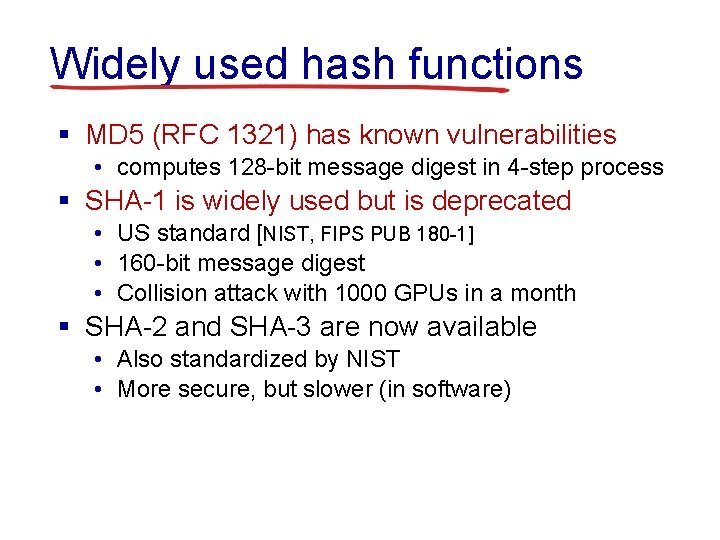Widely used hash functions § MD 5 (RFC 1321) has known vulnerabilities • computes 128 -bit message digest in 4 -step process § SHA-1 is widely used but is deprecated • US standard [NIST, FIPS PUB 180 -1] • 160 -bit message digest • Collision attack with 1000 GPUs in a month § SHA-2 and SHA-3 are now available • Also standardized by NIST • More secure, but slower (in software)Before You Go On a sheet of paper, answer the following (ungraded) question (no names, please): What was the muddiest point in today’s class?